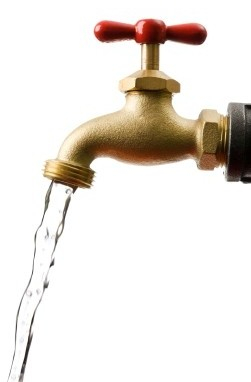# 8.3: Flow Rate

$$\newcommand{\vecs}{\overset { \rightharpoonup} {\mathbf{#1}} }$$ $$\newcommand{\vecd}{\overset{-\!-\!\rightharpoonup}{\vphantom{a}\smash {#1}}}$$$$\newcommand{\id}{\mathrm{id}}$$ $$\newcommand{\Span}{\mathrm{span}}$$ $$\newcommand{\kernel}{\mathrm{null}\,}$$ $$\newcommand{\range}{\mathrm{range}\,}$$ $$\newcommand{\RealPart}{\mathrm{Re}}$$ $$\newcommand{\ImaginaryPart}{\mathrm{Im}}$$ $$\newcommand{\Argument}{\mathrm{Arg}}$$ $$\newcommand{\norm}{\| #1 \|}$$ $$\newcommand{\inner}{\langle #1, #2 \rangle}$$ $$\newcommand{\Span}{\mathrm{span}}$$ $$\newcommand{\id}{\mathrm{id}}$$ $$\newcommand{\Span}{\mathrm{span}}$$ $$\newcommand{\kernel}{\mathrm{null}\,}$$ $$\newcommand{\range}{\mathrm{range}\,}$$ $$\newcommand{\RealPart}{\mathrm{Re}}$$ $$\newcommand{\ImaginaryPart}{\mathrm{Im}}$$ $$\newcommand{\Argument}{\mathrm{Arg}}$$ $$\newcommand{\norm}{\| #1 \|}$$ $$\newcommand{\inner}{\langle #1, #2 \rangle}$$ $$\newcommand{\Span}{\mathrm{span}}$$$$\newcommand{\AA}{\unicode[.8,0]{x212B}}$$

Flow rate is the measurement of a volume of liquid (i.e., water) which passes through a given cross-sectional area (i.e., pipe) per unit in time. In the waterworks industry, flow rates are expressed in several different units. The most common ones are shown below.

• Flow Rates
• cfs = cubic feet/second
• gpm = gallons/minute
• MGD = million gallons/dayFigure $$\PageIndex{1}$$

Depending on the application, flow rates are expressed in these or potentially other units. For example, the flow rate from a well or booster pump is commonly expressed as gpm, whereas annual production might be expressed as acre-feet per year (AFY). However, when solving a problem for flow rate the common unit of expression is cfs. The reason for this is in part due to the measurement of unit area of the structure that the water is passing through (i.e., pipe, culvert, aqueduct, etc.) The areas for these structures are typically expressed as square feet (ft2). In addition, the speed (distance over time) at which the water is flowing is commonly expressed as feet per second. The flow rate formula and how the units are expressed are shown in the example below.

• Flow Rate = Area x Velocity
• Flow Rate (Q) = Area (A) x Velocity (V)
• Q = A x V
• Q (cubic feet/sec) = Area (ft2) x Velocity (feet/sec)

Understanding flow rates and velocities can help with the design on pipe sizes for wells, pump stations, and treatment plants. With the understanding that velocities are typically in the range of 2 – 7 feet per second and the known flow rate, pipe diameters can be calculated. For example, if a new well is being drilled and the pump test data determines that the well can produce a specific flow, let’s say 1,500 gpm, and you do not want the velocity to exceed 6.5 fps, the required diameter of the pipe can be determined (see below).

### Example

Q = A x V or for this example A = Q/V since the flow rate (Q = 1,500 gpm) and velocity (V = 6.5 fps) are known.

The first step is to make sure the “known” values are in the correct units.

Velocity given at 6.5 fps is in the correct unit. However, the flow rate given in gpm needs to be converted to cfs.

• 1,500 gpm ÷ 448.8 = 3.34 cfs

Now that both values are in their correct unit, divide the two to get the unknown value, in this case, the Area (A).

• 3.34 cfs/6.5 fps = 0.51 ft2

Knowing that the Area is 0.51 ft2 and that the formula for Area is 0.785 x D2, the diameter of the pipe can be calculated.

• 0.51 ft2 = 0.785 x D2
• D2 = 0.51 ft/20.785
• D2 = 0.66 ft2

In order to find the value of the diameter (D), you must take the square root of D2.

• D2 = 0.66 ft2
• D = 0.81 ft

Since pipe diameters are typically expressed in inches, multiply the answer by 12.

• 0.81 ft x 12 in = 9.7 or 10 inches

## Exercises

Solve the following problems.

8.3: Flow Rate is shared under a not declared license and was authored, remixed, and/or curated by LibreTexts.